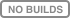# mlinsights - extensions to scikit-learn#mlinsights extends scikit-learn with a couple of new models, transformers, metrics, plotting. It provides new trainers such as QuantileLinearRegression which trains a linear regression with L1 norm non-linear correlation based on decision trees, or QuantileMLPRegressor a modification of scikit-learn’s MLPRegressor which trains a multi-layer perceptron with L1 norm. It also explores PredictableTSNE which trains a supervized model to replicate t-SNE results or a PiecewiseRegression which partitions the data before fitting a model on each bucket.

Function `pipeline2dot` converts a pipeline into a graph:

```from mlinsights.plotting import pipeline2dot
dot = pipeline2dot(clf, df)
```